+

# Breakeven Point

Author: Sophia Tutorial
##### Description:

Determine a firm's breakeven point on a graph.

(more)### Developing Effective Teams

*No strings attached. This college course is 100% free and is worth 1 semester credit.

37 Sophia partners guarantee credit transfer.

299 Institutions have accepted or given pre-approval for credit transfer.

* The American Council on Education's College Credit Recommendation Service (ACE Credit®) has evaluated and recommended college credit for 32 of Sophia’s online courses. Many different colleges and universities consider ACE CREDIT recommendations in determining the applicability to their course and degree programs.

Tutorial
what's covered
This tutorial will discuss the breakeven point and how firms will break even when total revenue equals total cost.

Our discussion breaks down as follows:

1. Profit/Loss
2. Short Run Profit to Long Run Adjustment
3. Short Run Loss to Long Run Adjustment
4. Zero Economic Profit
5. Profits in Less Competitive Industries

## 1. Profit/Loss

We know that profit equals revenues minus cost.

Profit = Revenues - Costs

If total revenue is greater than total cost (TR > TC), then the firm makes a profit.

If total revenue is less than total cost (TR < TC), then the firm sustains a loss.

If total revenue equals total cost (TR = TC), then the firm breaks even.

This last statement will be our focus in today's lesson.

Here is a graph that shows price and quantity on its axes for an apple farmer.

It shows marginal revenue, which is also our demand curve and price for a perfect competitor.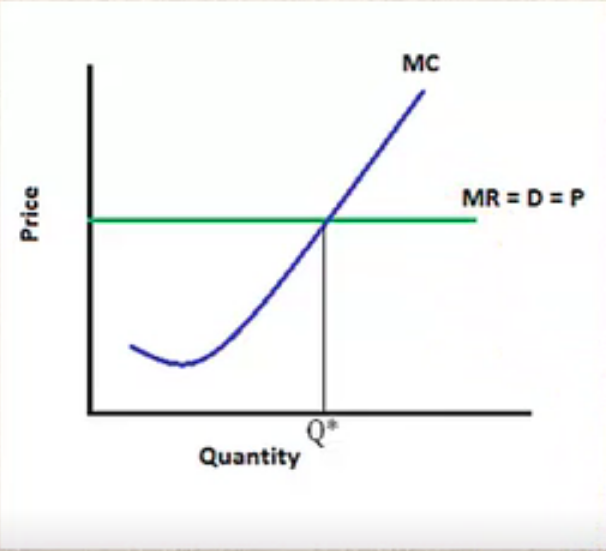Now, the first thing we do is find the profit-maximizing quantity, and we do this by equating marginal revenue and marginal cost.

You can see the gray line marking the equilibrium quantity or quantity that will maximize profit or minimize loss.

This is the best case situation, or the quantity that they will always produce.

Now we need an average total cost curve to compare to price to see if the firm is making a profit or loss.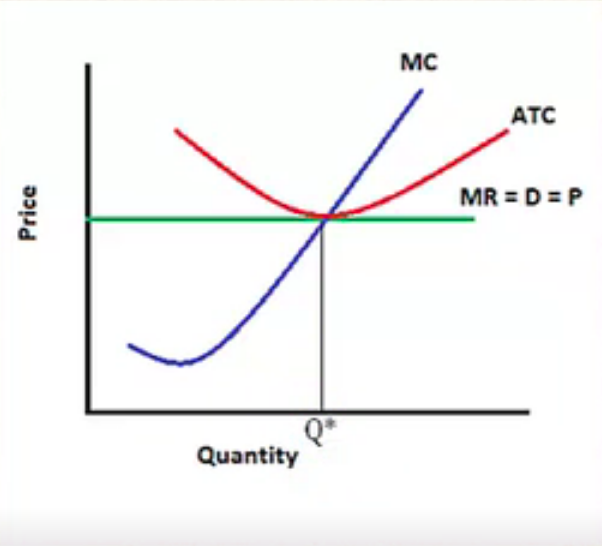It is drawn so that our price is exactly right where our average total costs are.

This means that on average, the price they are receiving is the same as the cost to produce each unit.

This graph shows that this is a breakeven situation, or zero profit. They are basically just covering costs.

Breakeven is a situation where there is no profit or loss.

term to know
Breakeven
A situation where there is no profit or loss

## 2. Short Run Profit to Long Run Adjustment

Next, let's discuss what is going to happen in the short run versus the long run.

Remember, the short run is a point in which a firm cannot adjust output and expenses in preparation to minimize cost per unit.

Something is fixed in the short run that firms can't do much about; there are more constraints in the short run.

Let's look at a graph of a short run profit situation.

Notice that we drew average total cost lower this time, below the point where marginal revenue equals marginal cost.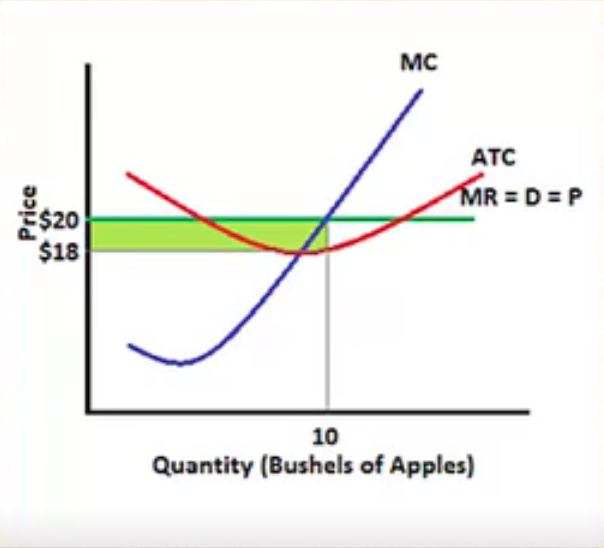This apple farmer is going to maximize his profit by producing 10, and the price he receives is \$20.

hint
Remember, he's a price-taker. This is why the demand curve is straight across and is the same as marginal revenue and price.

You can see that the price he receives, at \$20, is above his cost per unit, which is \$18. His total revenue is greater than total cost, and his price is greater than average total cost (per unit).

hint
Regardless of if you are looking at it per unit versus in total, it will result in the same thing.

In the short run, it is possible for perfect competitors to make a profit.

In perfect competition, there are no barriers to entry, so other firms will enter the market to get in on the profit opportunity.

So, what is going to happen now?

Well, now that more people have joined the industry, there is more competition.

When the industry supply curve shifts to the right and there is more competition, market price falls.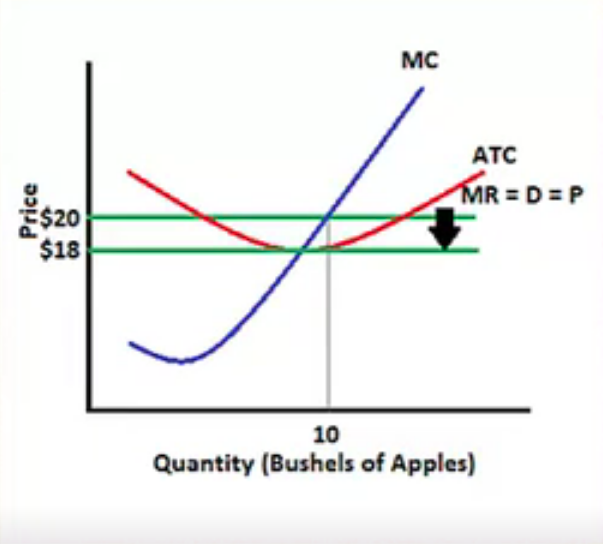As consumers, we like more competition because it means that prices fall.

However, look at the impact on the farmer. The price that he has to take now--he's a price-taker--fell down to \$18.

This is where our average total cost curve is now going to come in--right around \$18--so our economic profits disappear.

The price will fall until firms are breaking even, where price equals average total cost.

The long run, then, is a point in which the firm can adjust output and expenses to minimize cost per unit.

In the long run, firms can enter and exit.

terms to know
Short Run
A point in which a firm cannot adjust output and expenses in preparation to minimize cost per unit
Long Run
A point in which a firm can adjust output and expenses to minimize cost per unit

## 3. Short Run Loss to Long Run Adjustment

Now let's look at the short run loss situation.

In the short run, this firm is experiencing a loss. They are producing where marginal revenue equals marginal cost.

Notice that cost is now above, so price is less than average total cost, and they are losing \$5 per unit.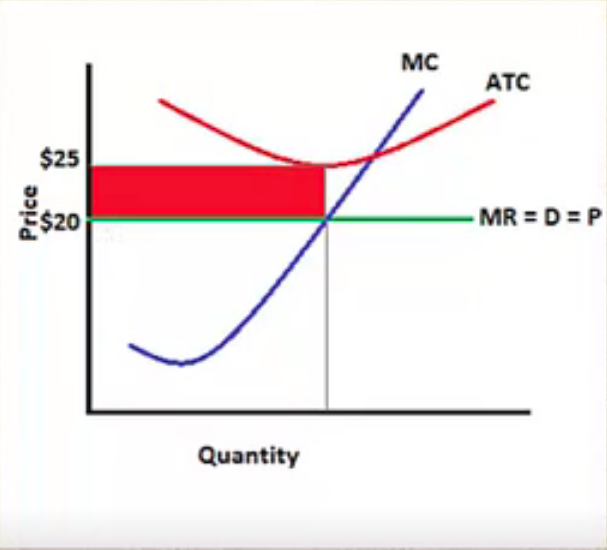There are no barriers to exit, so some of these farmers will exit the market.

Now that there is less competition in the industry, the market price will rise. Economic losses disappear, and price will rise until firms are breaking even, where price equals average total cost again.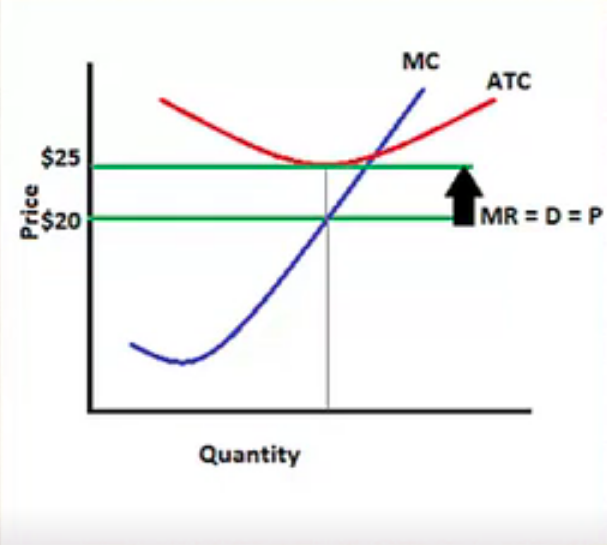## 4. Zero Economic Profit

Next, let's discuss what this zero economic profit means.

Economic profit, you may recall, is total revenue minus total costs, which include explicit costs and implicit, or opportunity, costs.

So, why would a firm ever continue to operate in the long run if they are earning zero economic profit? Remember, in the long run, we just stated that perfectly competitive firms earn zero economic profit in the long run.

Well, remember that this includes those firms covering their opportunity cost. Therefore, zero economic profit--or breaking even--in economics means that they may still be earning an accounting profit.

EXAMPLE

For example, the apple farmer is earning enough returns that they are the same as his next best alternative, or what he gave up. He is not making an economic profit, which means he is not doing better than what he has given up--but he's not doing worse, either. He is not earning economic losses, so it is just enough to keep the apple farmer in the industry.

This is long run equilibrium for perfect competition simply because where there are profits, there are no barriers to entry, so firms will enter.

Where there are losses being seen, some firms are going to exit.

This is why it's not equilibrium yet, because there is change occurring.

Keep in mind, though, that this is a theoretical concept because markets change rapidly all the time.

There are always new advertisements or products being recalled; factors extraneous to what we have been looking at in these simplified models happen constantly.

Therefore, is equilibrium ever completely able to be reached? Maybe, maybe not. It is important to keep in mind that we are talking about this as a theoretical concept.

term to know
Economic Profit
Total revenue calculated minus explicit costs and opportunity costs

## 5. Profits in Less Competitive Industries

Finally, it is important to understand that profits are sustainable in other industries.

In less competitive industries than perfect competition, there are barriers to entry.

EXAMPLE

For instance, if the airline industry is making huge profits, these barriers to entry do exist, because it is so expensive to get into this particular market. It is not possible for a large amount of producers to suddenly enter to compete with them and lower price.

Therefore, the less competitive the industry, usually because there are more barriers to entry, the more capable the firms are of realizing profits in the long run.

summary
We began today's lesson by finding profit and loss on a graph. We learned that firms break even when total revenue equals total cost. However, if we look at it on a per unit basis, where price equals average total cost, we learned that perfectly competitive firms earn zero economic profit in the long run, because short run profit causes entry into the industry, while short run losses cause exit out of the industry.

We learned that less competitive firms (imperfectly competitive) can keep sustaining those profits in the long run because of barriers to entry. Lastly, keep in mind that zero economic profit does not mean zero accounting profit, because it means that those firms are, in fact, covering their opportunity costs or what was given up.

Source: Adapted from Sophia instructor Kate Eskra.

Terms to Know
Breakeven

A situation where there is no profit or loss.

Economic Profit

Total revenue calculated minus explicit costs and opportunity costs.

Long-Run

A point in which a firm can adjust output and expenses to minimize cost per unit.

Short-Run

A point in which a firm cannot adjust output and expenses in preparation to minimize cost per unit.

Rating